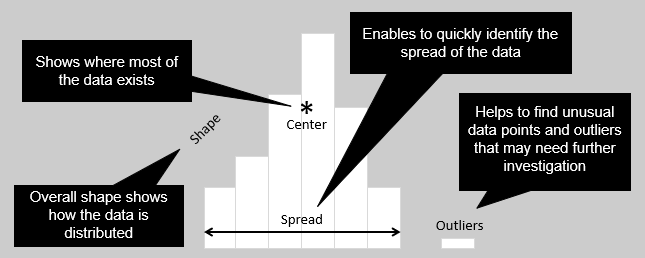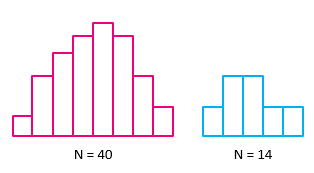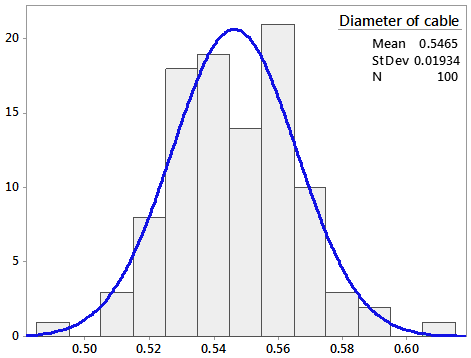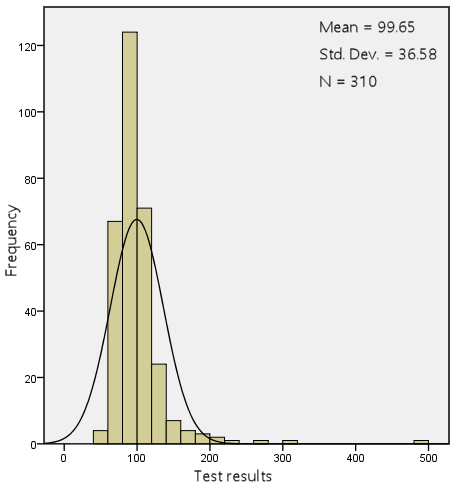By the CIToolkit content team   |   3 minutes read

# Histogramhistogram is a graph which shows the frequency of continuous data values. It is a type of bar chart that can be drawn either vertically or horizontally. Histograms are widely used in statistics, process improvement, scientific research, economics, and in social and human sciences.

Histograms are mainly used to explore data as well as to present the data in an easy and understandable manner. They are often used as the first step to determine the underlying probability distribution of a data set or a sample. They allow to visually assess the shape of the distribution, the central tendency, the amount of variation in the data, as well as the presence of gaps, outliers or unusual data points.Histograms can be helpful to identify whether you can apply certain statistical tests to perform potential improvement opportunities. Additionally, you can verify whether an improvement has been achieved by exploring the histogram of the data before and after the improvement initiative. Histograms can also be helpful to identify whether variability is within specification limits, whether the process is capable, and whether there is a shift in the process over time.Histograms are ideal to represent moderate to large amount of data. In practice, a sample size of at least 30 data values would be sufficient. A histogram may not accurately display the distribution shape if the data size is too small. Dot plots are preferred over histograms when representing small amount of data.

## How to Construct a Histogram Manually

Note: There are many applications and online services that allow the creation of histograms quickly and automatically (such as Minitab). However, you may construct a histogram manually by following the below steps:

• Collect the data set and prepare it for the analysis.
• Draw a horizontal line and divide it into equal intervals or bins (10 intervals for example). The total width should be equal to the range of the data.
• Draw bars above each bin to represent the frequency of the data values within each interval. The bars should be adjacent with no gaps between them.
• Indicate the mean of the data and other important information (such as the standard deviation and the specification limits).

## Example

The following is a histogram that represents the distribution of cable diameters in a manufacturing process.The above chart shows the results of a data set that belongs to Minitab Inc.

The result can be summarized using day to day language such as: “The distribution looks symmetric around the cable diameter mean (0.546 cm) and appears to fit the Normal Distribution fairly well”.

## Example

The histogram below illustrates an analysis that was conducted for diagnosing the presence of diabetes at a workplace.The distribution of the data is skewed to the right. It is more like an exponential distribution which is normal for this type of data.

There are many tools that can help you to create histograms. One of the simplest ways is to use this template.

Other Formats

Related Articles

Related Templates